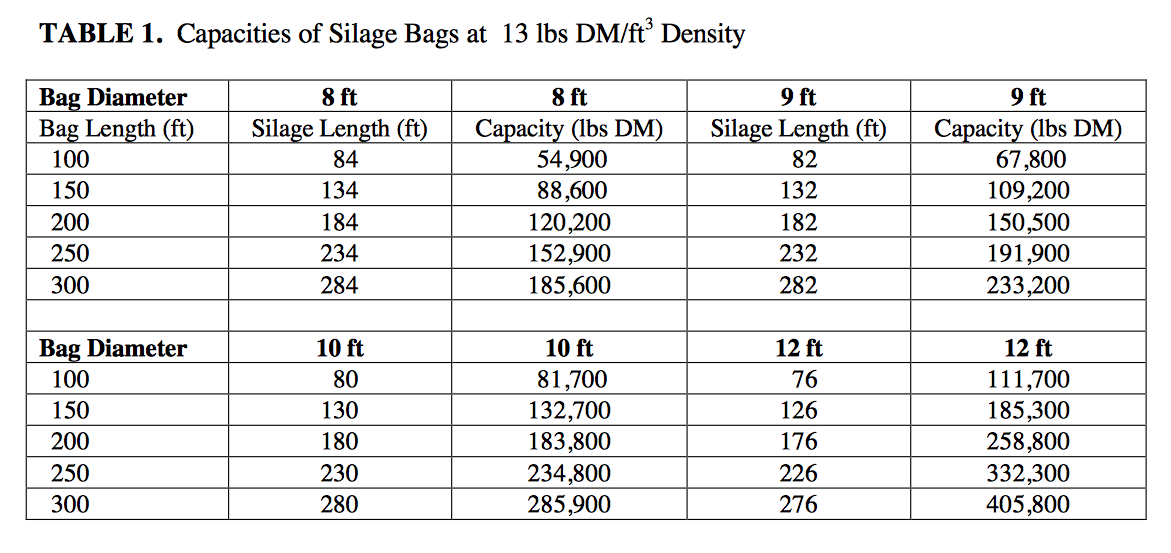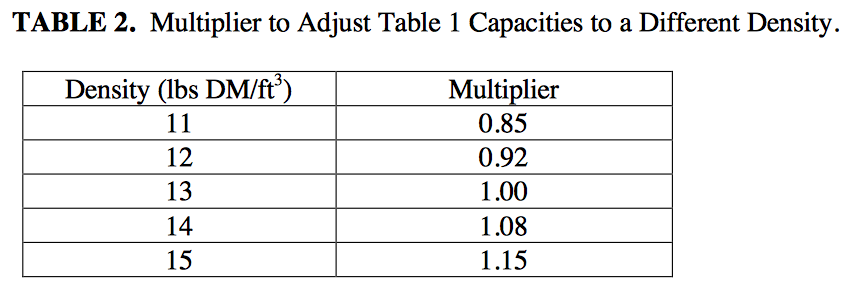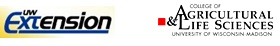# Silage Bag Capacity# Silage Bag Capacity

by Brian J. Holmes

We frequently get questions about the amount of silage in a silo bag. One way to estimate this value is to calculate the volume in the bag and multiply by its density. The volume of a round bag is calculated as:

V = 3.14 × (D÷ 4) × L

Where:       V  =  Volume (ft3)
spacespaciiD  =  Diameter (ft)
spacespaceL  =  Length of Silage (ft)

When full length bags are used, the length of silage is the bag length minus the unused portion needed to seal each end of the bag.

The quantity of dry matter in the bag is the volume multiplied times the dry matter density. The dry matter density can vary from bag to bag and is based on machine type and adjustment as well as forage type. Typical densities range between 11-15 lbs DM/ft3. Table 1 has been developed to show silo bag capacity based on the following assumptions.

Round Bags
Silage Length  =  Bag Length − (2 × Diameter)
Density  =  13 lbs DM/ft3Use the multiplier in Table 2 to adjust the values in Table 1 for a different density. For example, the quantity of silage in a 200′ × 9′ bag packed to 15 lbs DM/ft3 is:

150,500 lbs DM × 1.15  =  173,100 lbs DMTable 1 lists dry matter in one bag. If you need to know the capacity in lbs of silage as fed, divide the table value by the dry matter content. For example, 65% moisture silage in a 200-foot long bag of 9 ft diameter weighs:

430,000 lbs AF  =  150,500 lbs DM/0.35

when packed at 13 lbs DM/ft3 density. Divide this value by 2000 lbs/T to obtain 215 TAF.Biological Systems Engineering Department
College of Agricultural and Life Sciences